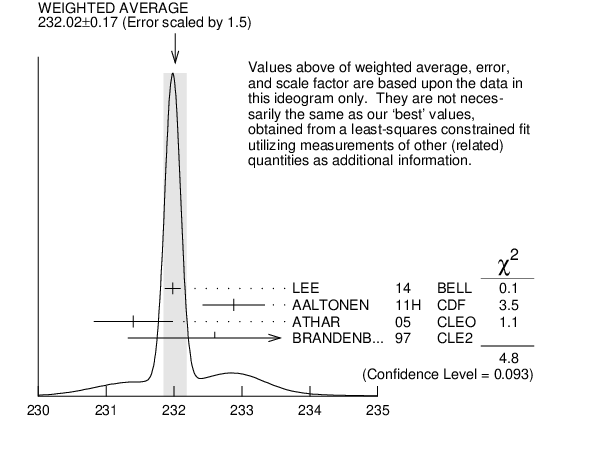# ${\boldsymbol m}_{{{\boldsymbol \Sigma}_{{c}}{(2520)}^{0}}}–{\boldsymbol m}_{{{\boldsymbol \Lambda}_{{c}}^{+}}}$ INSPIRE search

VALUE (MeV) EVTS DOCUMENT ID TECN  COMMENT
$\bf{ 232.02 {}^{+0.15}_{-0.14}}$ OUR FIT  Error includes scale factor of 1.3.
$\bf{ 232.02 \pm0.17}$ OUR AVERAGE  Error includes scale factor of 1.5.
$231.98$ $\pm0.11$ $\pm0.04$ 41k
 2014
BELL ${{\mathit e}^{+}}{{\mathit e}^{-}}$ at ${{\mathit \Upsilon}{(4S)}}$
$232.88$ $\pm0.43$ $\pm0.16$ 9.0k
 2011 H
CDF ${{\mathit p}}{{\overline{\mathit p}}}$ at 1.96 TeV
$231.4$ $\pm0.5$ $\pm0.3$ 1.3k
 2005
CLEO ${{\mathit e}^{+}}{{\mathit e}^{-}}$ , $9.4 - 11.5$ GeV
$232.6$ $\pm1.0$ $\pm0.8$ 504
 1997
CLE2 ${{\mathit e}^{+}}{{\mathit e}^{-}}$ $\approx{}{{\mathit \Upsilon}{(4S)}}$${\mathit m}_{{{\mathit \Sigma}_{{c}}{(2520)}^{0}}}–{\mathit m}_{{{\mathit \Lambda}_{{c}}^{+}}}$ (MeV)
References:
 LEE 2014
PR D89 091102 Measurements of the Masses and Widths of the and Baryons
 AALTONEN 2011H
PR D84 012003 Measurements of the Properties of ${{\mathit \Lambda}_{{c}}{(2595)}}$, ${{\mathit \Lambda}_{{c}}{(2625)}}$, ${{\mathit \Sigma}_{{c}}{(2455)}}$, and ${{\mathit \Sigma}_{{c}}{(2520)}}$ Baryons
 ATHAR 2005
PR D71 051101 New Measurement of the Masses and Widths of the ${{\mathit \Sigma}_{{c}}^{*++}}$ and ${{\mathit \Sigma}_{{c}}^{*0}}$ Charmed Baryons
 BRANDENBURG 1997
PRL 78 2304 Observation of Two Excited Charmed Baryons Decaying into ${{\mathit \Lambda}_{{c}}^{+}}{{\mathit \pi}^{\pm}}$